﻿ Static Condensation – FEM Analysis | Aerospace Engineering

## Static Condensation – FEM Analysis

• Background Theory

With “condensation” is indicated a procedure adopted for the structural analysis to carry out a matrix of stiffness and load boundary conditions of a detailed finite model (DFEM), starting from a raw global finite model (GFEM). The DFEM represents a detailed and more accurate version of limited GFEM area for which the structural analysis has to be performed in order to obtain more accurate results. A basic objective of a structural survey is to verify that the finite element model (FEM) of a structure is sufficiently accurate to predict the structure’s response to operating environments. In addition to a static survey, a modal survey is recommended in order to predict the  structure’s response to operating environments in sufficiently accurate manner. Many methods for reducing FEM matrices are currently in use. The simplest procedure uses the Guyan reduction method. This approach assumes that the interpolation shapes can be calculated using the FEM  stiffness matrix to solve a static solution of the form:Where subscripts “a” represents the degrees of freedom to be kept in the reduced model and “d” represents the degrees of freedom to be condensed out (omitted degrees of freedom). The dependent degrees of freedom,xd, can be expressed in terms of indipendend degrees of freedom,xa , by solving the upper partition of the Eq. (1):Therefore:Where [Iaa] is the identical matrix, and: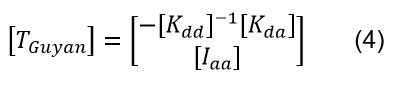is the static transformation matrix. The main assumption of Guyan reduction is that there are no forces applied on the omitted degrees of freedom, therefore Eq.(4) can be reduced:The reduced stiffness and mass matrices can be formed using the original FEM matrices and the transformation matrix T_Guyan:The Guyan reduction method produces an exact reduction on the stiffness if no forces are applied on the omitted degrees of freedom. If this is not the case, then the Guyan reduction is only approximate. The improved reduced system (IRS) proposes an algorithm to correct this approximation in a static analysis by including the second term of Eq.(3). The procedure to derive the correction term is obtained by first formulating the standard eigenvalue equation: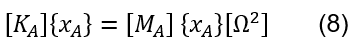Using the Guyan transformation matrix shown in Eq.(4), Eq.(8) can be reduced, and by successive transformations the IRS transformation matrix can be derived by: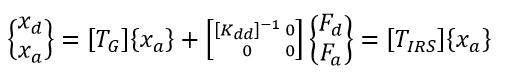where: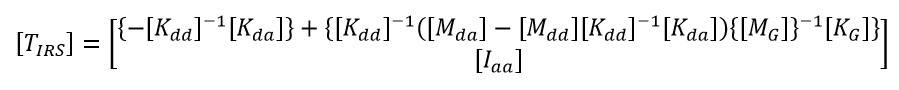• Procedure using MSC Nastran
1. Groups of DFEM/GFEM
2. Input Data for Condensation
3. Nastran Run

Note:

• The condensation of Nastran GFEM (stiffness + external load), when appropriate, is a convenient way of decreasing the size of a problem
• It can be fully validated within the Nastran GFEM environment
•  It can be used with Abaqus, by converting the stiffness matrix and the external load vector to Abaqus format
• This technique requires a particular care, especially when some boundary nodes in the GFEM are some degrees of freedom involved in couplings, and when some external loads are applied to some boundary nodes

• 1) Groups of DFEM/GFEM

The figure below shows the GFEM . The red colour area will be condensed, the cyan colour area will be substituted by the DFEM.

The figure below shows the nodes and elements of the GFEM which will be replaced by the DFEM.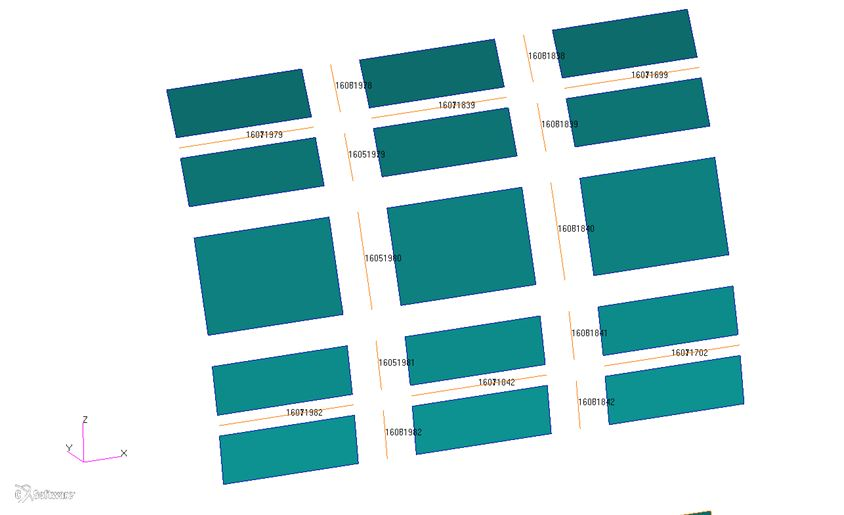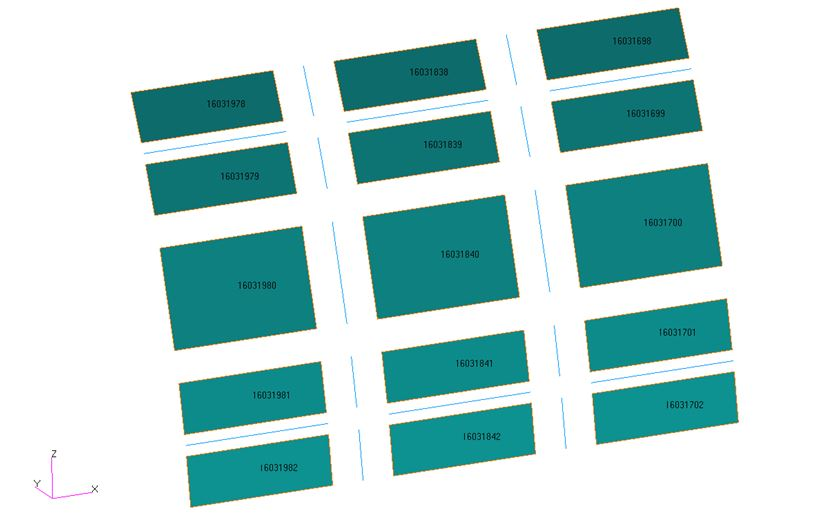Figure 2: nodes and elements of the GFEM which will be replaced by the DFEM

The figure below shows the DFEM (it’s a Window), the detailed finite element model related to the GFEM area reported above. This DFEM will be combined with the KAAX and PAX.

Fort he GFEM, create a group called DFEM_Area which contains all elements, MPCs and nodes which will be replaced by the DFEM.
This group must not contain the interface nodes between GFEM and DFEM. The interface nodes are the connection between DFEM and GFEM and remain in the GFEM.

Create a group called DFEM_Area_Nodes . This is the same as DFEM_Area group but contains Nodes only.

Create a group called Interface_Nodes .
This group contains all nodes which connect the DFEM and the GFEM, for example skin, stinger, frames, crossbeams, z-stut connection, floor-struts, x-paddles, hinge, latch-fittings, etc. Create a group called GFEM_DFEM_Area which is the summation of groups DFEM_Area and Interface_Nodes .

Export the group GFEM_DFEM_Area using the “model only” option.
Filename: GFEM_DFEM_Area.dat

Export the group DFEM_Area to a Nastran Set
(Utilities – Groups – P3 groups to MSC Nastran Sets)
Filename: DFEM_Area.set

Export the group Interface_Nodes to a Nastran Set.
Filename: Interface_Nodes.set

• 2) Input-Data for the Condensation-run

To get the Nastran input for the condensation-run copy the GFEM-file and apply the following steps on the copy:

• Step 1: Comment out all elements, MPCs and nodes which can be found in DFEM_Area.set. All cards referring to the commented out nodes ,e.g. TEMP- and FORCE-cards, must be commented out, too.
• Step 2:  Add the ASET1 cards to the Bulk Data Section: All nods which can be found in Interface_Nodes.set must be part of an ASET1 card. Usually the ASET1-card contains all six degrees of freedom (DOF). If some DOFs are already part of another set (e.g. via SPC), these DOFs must not be part of the ASET1 card.

Example:

BEGIN BULK
\$
\$ ASET1 Nodes at boundaries to DFEM Surround
\$
\$–1—><–2—><–3—><–4—><–5—><–6—><–7—><–8—><–9—>
ASET1 1234 1601698 1601699 1601700 1601701 1601702 1601703 1601838
1601843 1601978 1601983 1602118 1602119 1602120 1602121 1602122
1602122
\$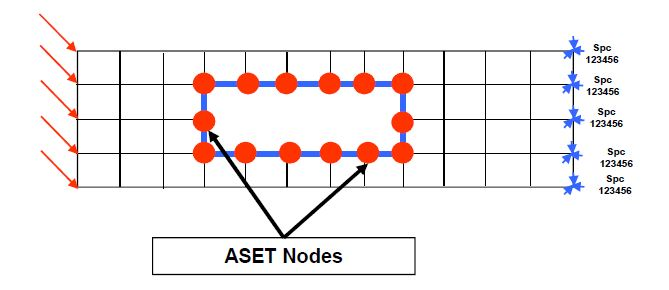This model will be the model to condense ( GFEM model without the reduced local model ). ASET nodes are the condensation nodes, the condensed model will include the local model frontier and in this example, It will include boundary conditions (constraints + loading ).

Set of boundary nodes have to take care of the following points:

1.Choose the DFEM model boundary far from the studied area: the size of this area is of the order of magnitude of the GFEM element width at the boundary (Example: for a fuselage: 3 stringer pitches in the circumferential direction, and 1 frame pitch in the longitudinal direction)

2.The boundary nodes should not be constrained:

– No constraint node

– Boundary nodes should not be dependent in kinematic or distributing couplings

• Step 3:  Add the EXTSEOUT statement to the Case Control Section just before the SUBCASE-statements. Example:

\$
SUBCASE 1501
LABEL=ZCT Ultimate
SUBCASE 1502
LABEL=DT1.15 Limit

• 3) Nastran-Run

Perform a Nastran run, and afterwards get from the punch file the KAAX and PAX (DMIG Matrix) and save them in separate files.

– KAAX starts at keyword KAAX and ends one line above keyword PAX.

– PAX starts at keyword PAX and ends one line above keyword VAX:

Example: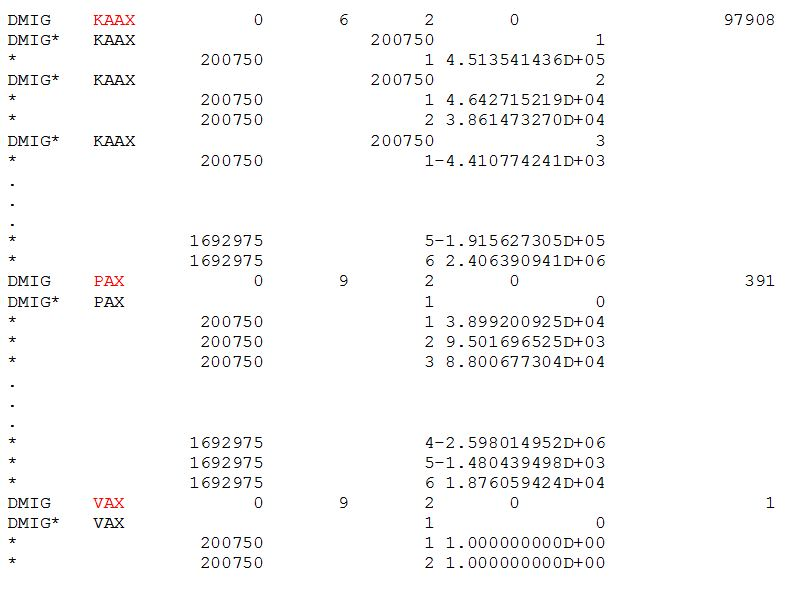If you perform the condensation for several load cases (e.g. flight, with temperature, …) the KAAX has to be the same for all runs (dowload an example  here).

Once the condensed stiffness and loads are obtained, as describe then are applied on the reduce model (DFEM)..

This punch file with the KAAX and PAX matrix has to be included in the final DFEM (without any loads or SPC), and with the keywords KAAX and PAX (highlighted below):

 –  GFEM Boundary nodes have to be imported in the DFEM (see picture beside)   – Some couplings have to be defined between DFEM nodes and GFEM nodes, in order to ensure a satisfying continuity in displacements and internal loads across the boundary   –  In Nastran, the techniques used to define these couplings is the mesh sewing method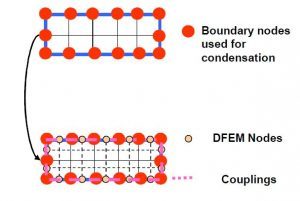Note:
1) Interface Nodes-aset nodes- must have same coordinate system than global FEM
2) No constraint data is given. The local model is constrained by reduced stiffness matrix . If constraints and/or external forces are applied on local model, they have to be added in the local model nastran file(SPC1 data, FORCE data, MOMENT data, etc..)

• Mesh Sewing Technique

For DFEM/GFEM connection purpose, the mesh sewing method is recommended.

 Use of RBE3/MPC distributing coupling along the 6 DOFs (barycentric equations, the coefficients of the kinematic relations take into account the geometrical positions of the slave nodes with respect to the master nodes).
Each mesh sewing node must tend to be perfectly aligned with GFEM nodes. If the tolerance is too high, the quality of the rigid modes will be altered.
 These relations are explicit relations: if some nodes have to be moved, the relations must be updated.
Check that the slave nodes of the kinematic relation are not involved in another relation (RBE2 or MPC for example) and, if this is not the case, check the sequencing of relations.

An area of altered stress distribution in the DFEM is present at the mesh sewing level, the size of this area is the same order of magnitude of the GFEM element width. Recommendation: put the mesh sewing border away from the area of interest, at least at a distance equal to a GFEM element width. For each slave node 2 master nodes bracing it (by 2 RBE3s). Below is depicted a scheme of connection between the slave node (E) and the 2 master nodes (M1, M2).Between these 3 nodes, a barycentric relation has to be considered. The expression for one DOF is the following:To have valid rigid modes and a valid transition, each couple (2 master nodes, 1 slave node) must be aligned. Below is shown a connection setting of 2 RBE3s (done in MSC Patran) between Master nodes (2300840, 2300830), and a Slave node (3167). The first RBE3 take in to account translations as DOFs (Ux, Uy, Uz) for indipendent terms, the second one the rotations (Rx, Ry, Rz).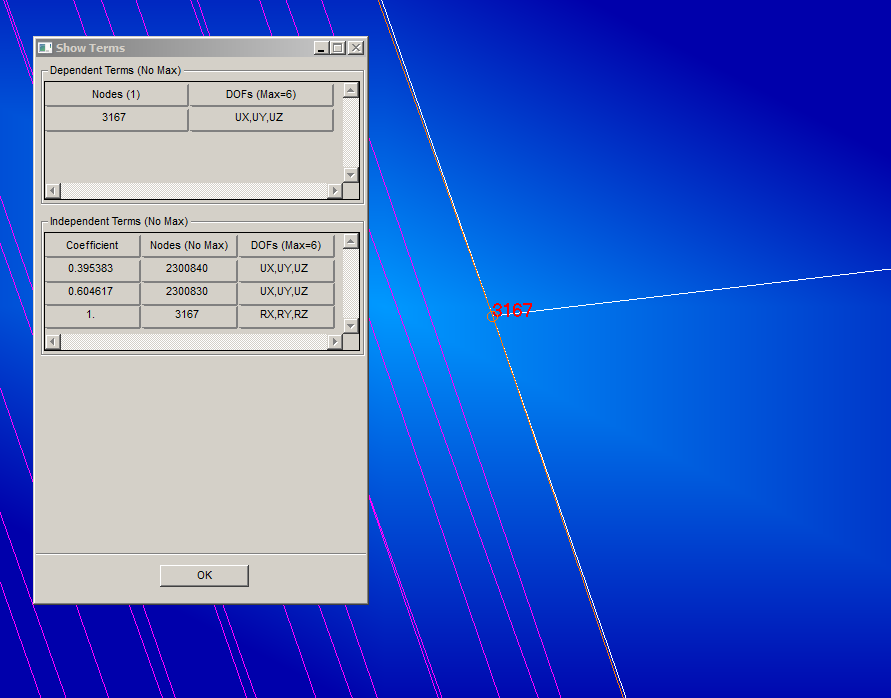• 4) Validation of Condensation

The condensation process has to be checked in order to ensure the correctness of procedure and results. As first step is recommended to compared the displacement-plots obtained from DFEM with KAAX/PAX matrix obtained by the procedure descripted above, with the GFEM area corresponded to DFEM embedded in the same KAAX and PAX matrices. The OLOAD comparison also is recommended (in Nastran the output request is: OLOAD(PLOT)=ALL). The comparison normally is done for three different flight load cases: a pure mechanical one, a mechanical load case with temperature and the 2x internal pressure load case. Below is reported an example of comparison:If there is no difference in the translational OLODs and only very small differences in the rotatory OLOADs it’s assumed that the loading of the DFEM is correct.